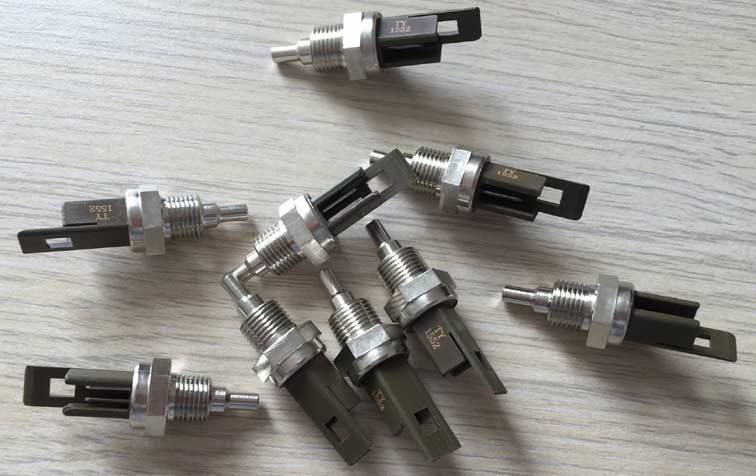﻿ What is digital temperature sensor?_

China sensor manufacturers

# What is digital temperature sensor?

Digital temperature sensor and the traditional thermistor is different. Digital temperature sensors use integrated chips, Using single-bus technology, It can effectively reduce the external interference, Improve the accuracy of the measurement. At the same time, it can be directly measured temperature into a serial digital signal for microcomputer processing, Interface is simple, So that data transmission and processing to simplify.

The digital temperature sensor can be connected directly to the microcontroller,Saving design time, PCB area and cost. They can be flexible to reduce current consumption, This is particularly useful for battery-powered applications. The user can also program the temperature limit value (the THIGH and TLOW), the demand for alarm. If the programmed limit is exceeded,Can be interrupted, so that the microcontroller to operate. Many IC design systems in order to save board size and reduce costs, Integrate ADC and DAC into a single chip. Some also have built-in algorithms, Used to consume the maximum power and keep the noise at least when driving the fan at the optimum speed.

The digital temperature sensor is based on the following principles:
The diode-connected transistor base-emitter voltage VBE is proportional to its temperature, In the operating temperature range,VBE exhibits a negative temperature coefficient of about -2 mV / ° C. In fact, the absolute value of VBE varies with the transistor. To make these changes zero, The circuit must calibrate each transistor. The general solution to this problem is to compare the transistor ΔVBE when two different current densities are applied to the transistor emitter (see Figure 1).
Use the following formula to find the temperature:
(1) with I1 = NI2 into the above formula,
(2) where N: I1 / I2 current ratio,
K: Boltzmann constant,
q: charge,
T: Kelvin absolute temperature.
N, K, and q are known constants,
So that: ΔVBE = (constant) (T) or T = (constant) (ΔVBE) is placed in the bias diode of the transistor to prevent noise from the measurement interference. The maximum ΔVBE from the sensing transistor is tens of millivolts, Therefore, the need for effective processing of the pole pair signal to achieve ADC requirements. Gain level with switched capacitor circuit and chopper amplifier. The ADC converts the analog signal proportional to the absolute temperature to a digital word (expressed in ° C).﻿
﻿